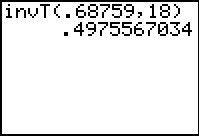Knowledge Base

Solution 11717: Calculating the Inverse Student-T (invT) Probability on the TI-84 Plus Family or TI-Nspire™ Handheld in TI-84 Plus Mode.

How can I calculate the inverse student-T probability on the TI-84 Plus family or TI-Nspire handheld in TI-84 Plus mode with OS 2.30 and higher?

The Inverse Student-T is usually used for determining probabilities of population means from sampled distributions when the standard deviation of the population is unknown. Specifically, t = (x-u)*sqrt(n)/s with n-1 degrees of freedom and where x is the sampled mean, u is the population mean, s is the sample standard deviation, n is the sample size, and the population is Gaussian.

Example: The command for this function is under the DISTR menu. Please refer to the instructions provided below.

1) Press [2nd][DISTR]
2) Select 4:InvT(
3) Enter a scalar or list of values at which to evaluate the t inverse and a scalar value for degrees of freedom separated with a comma. ' We will key in the following example: InvT(0.68759,18)
4) Press [ENTER] to display the answer of 0.4975567034